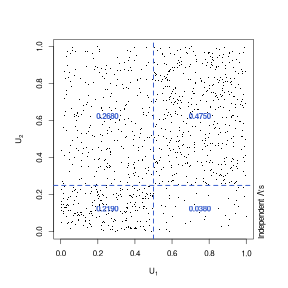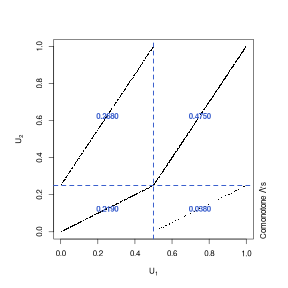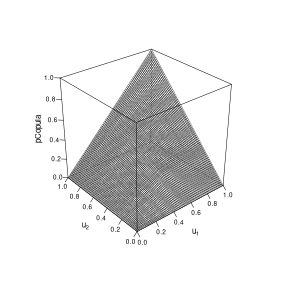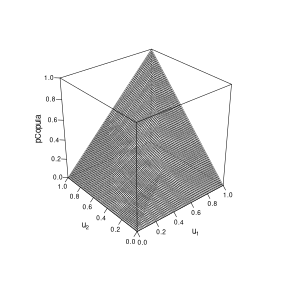# Exploring Empirical Copulas

#### 2023-01-20

library(copula)

## 1 Auxiliary functions

The following is merely an auxiliary for computing a log-density later.

##' @title Compute log(x_1 + .. + x_n) for given log(x_1),..,log(x_n)
##' @param lx n-vector containing log(x_1),..,log(x_n)
##' @author Marius Hofert
lsum <- function(lx) {
l.off <- max(lx)
l.off + log(sum(exp(lx - l.off)))
}

The next function implements, in pure R, various empirical copula estimators. The code is rather of pedagogical nature than optimized for speed (and copula mostly uses C code for the evaluation).

##' @title Evaluate Empirical Copulas
##' @param u evaluation points (in [0,1]^d)
##' @param U points (in [0,1]^d) based on which the empirical copula is built
##' @param smoothing character string indicating the smoothing method
##' @param offset shift when computing the outer sum (over i)
##' @param log logical indicating whether the log-density is to be returned
##'        (only applies to smoothing = "dbeta")
##' @param ... additional arguments passed to the underlying rank()
##' @return empirical copula values
##' @author Marius Hofert
##' @note See Hofert et al. (2018, "Elements of copula modeling with R")
empirical_copula <- function(u, U, smoothing = c("none", "pbeta", "dbeta", "checkerboard"),
offset = 0, log = FALSE, ...)
{
stopifnot(0 <= u, u <= 1, 0 <= U, U <= 1)
if(!is.matrix(u)) u <- rbind(u)
if(!is.matrix(U)) U <- rbind(U)
m <- nrow(u) # number of evaluation points
n <- nrow(U) # number of points based on which the empirical copula is computed
d <- ncol(U) # dimension
stopifnot(ncol(u) == d)
R <- apply(U, 2, rank, ...) # (n, d)-matrix of ranks
switch(match.arg(smoothing),
"none" = {
R. <- t(R) / (n + 1) # (d, n)-matrix
vapply(seq_len(m), function(k) # iterate over rows k of u
sum(colSums(R. <= u[k,]) == d) / (n + offset), NA_real_)
},
"pbeta" = {
## Note: pbeta(q, shape1, shape2) is vectorized in the following sense:
##       1) pbeta(c(0.8, 0.6), shape1 = 1, shape2 = 1) => 2-vector (as expected)
##       2) pbeta(0.8, shape1 = 1:4, shape2 = 1:4) => 4-vector (as expected)
##       3) pbeta(c(0.8, 0.6), shape1 = 1:2, shape2 = 1:2) => 2-vector (as expected)
##       4) pbeta(c(0.8, 0.6), shape1 = 1:4, shape2 = 1:4) => This is
##          equal to the recycled pbeta(c(0.8, 0.6, 0.8, 0.6), shape1 = 1:4, shape2 = 1:4)
vapply(seq_len(m), function(k) { # iterate over rows k of u
sum( # sum() over i
vapply(seq_len(n), function(i)
prod( pbeta(u[k,], shape1 = R[i,], shape2 = n + 1 - R[i,]) ), # prod() over j
NA_real_)) / (n + offset)
}, NA_real_)
},
"dbeta" = {
if(log) {
vapply(seq_len(m), function(k) { # iterate over rows k of u
lsum( # lsum() over i
vapply(seq_len(n), function(i) {
## k and i are fixed now
lx.k.i <- sum( dbeta(u[k,], shape1 = R[i,], shape2 = n + 1 - R[i,], log = TRUE) ) # log(prod()) = sum(log()) over j for fixed k and i
},
NA_real_)) - log(n + offset)
}, NA_real_)
} else { # as for 'pbeta', just with dbeta()
vapply(seq_len(m), function(k) { # iterate over rows k of u
sum( # sum() over i
vapply(seq_len(n), function(i)
prod( dbeta(u[k,], shape1 = R[i,], shape2 = n + 1 - R[i,]) ), # prod() over j
NA_real_)) / (n + offset)
}, NA_real_)
}
},
"checkerboard" = {
R. <- t(R) # (d, n)-matrix
vapply(seq_len(m), function(k) # iterate over rows k of u
sum(apply(pmin(pmax(n * u[k,] - R. + 1, 0), 1), 2, prod)) / (n + offset),
NA_real_) # pmin(...) = (d, n)-matrix
},
stop("Wrong 'smoothing'"))
}

## 2 Checking the various (smoothed) empirical copulas

Let us first generate some copula samples based on which we then compute the empirical copulas.

## Generate copula data based on which to build empirical copula
n <- 1000 # sample size
d <- 2 # dimension
set.seed(271)
U <- rCopula(n, copula = gumbelCopula(iTau(gumbelCopula(), tau = 0.5), dim = d))

Next, consider a grid of evaluation points for the empirical copulas (and a density in one of the cases).

## Evaluation points
n.grid <- 26
sq <- seq(0, 1, length.out = n.grid)
u <- as.matrix(expand.grid("u" = sq, "u" = sq, KEEP.OUT.ATTRS = FALSE))
## ... for the density of the empirical beta copula
delta <- 0.01
sq. <- seq(delta, 1-delta, length.out = n.grid)
u. <- as.matrix(expand.grid("u" = sq., "u" = sq., KEEP.OUT.ATTRS = FALSE))

Now let us evaluate the empirical copula, the empirical beta copula, its density and the empirical checkerboard copula at u (for the density of the empirical beta copula, we use u.).

## Evaluate empirical copulas
emp.cop.none   <- empirical_copula(u,  U = U)
emp.cop.pbeta  <- empirical_copula(u,  U = U, smoothing = "pbeta")
emp.cop.dbeta  <- empirical_copula(u., U = U, smoothing = "dbeta")
lemp.cop.dbeta <- empirical_copula(u., U = U, smoothing = "dbeta", log = TRUE)
stopifnot(all.equal(lemp.cop.dbeta, log(emp.cop.dbeta))) # sanity check
emp.cop.chck   <- empirical_copula(u,  U = U, smoothing = "checkerboard")

## 3 Application to show non-uniqueness of Sklar’s Theorem for Bernoulli margins

We now consider a classical example based on two Bernoulli margins with failure probabilities $$1-p_1,1-p_2$$, respectively. In particular, according to the first part of Sklar’s Theorem, the copula is only uniquely determined in $$(1-p_1,1-p_2)$$ and we construct two different mass distributions which lead to the same copula value in $$(1-p_1,1-p_2)$$.

First, we generate dependent Bernoulli random variables.

## Generate some data with Bernoulli margins
tau <- 0.5
gc <- normalCopula(iTau(normalCopula(), tau = tau))
n <- 1e4  ## <-- use this  for scientific reason
n <- 1000 # <<< prefer to decrease *.html and final package size
set.seed(271)
U <- rCopula(n, copula = gc)
p <- c(1/2, 3/4) # marginal probabilities p_1, p_2 of being 1
stopifnot(0 < p, p < 1)
X <- sapply(1:2, function(j) qbinom(U[,j], size = 1, prob = p[j]))
mean(rowSums(X) == 0) # p = P(X_1 = 0, X_2 = 0) = C(1-p_1, 1-p_2)
##  0.219

We now proceed as in the (stochastic) proof of the first part of Sklar’s Theorem and consider the generalized distributional transforms of the two margins. To this end, we implement the modified distribution function of a Bernoulli distribution:

## Define generalized distributional transform (for one margin)
F <- function(X, Lambda, p)
{
is0 <- X == 0
res <- numeric(length(X))
res[is0]  <- Lambda[is0] * (1-p)
res[!is0] <- (1-p) + Lambda[!is0] * p
res
}

Based on, first, independent and, second, comonotone uniform random variables $$\Lambda$$ (as appearing in the generalized distributional transforms), we obtain two different mass distributions and thus copulas with equal values at $$(1-p_1,1-p_2)$$.

## Compute U's for two different Lambda
Lambda.Pi <- matrix(runif(n * 2), ncol = 2) # independence case for Lambda
Lambda.M <- cbind(Lambda.Pi[,1], Lambda.Pi[,1]) # comonotone case for Lambda
U.Pi <- sapply(1:2, function(j) F(X[,j], Lambda = Lambda.Pi[,j], p = p[j]))
U.M  <- sapply(1:2, function(j) F(X[,j], Lambda = Lambda.M [,j], p = p[j]))

As a basic test, one could visually check whether the mass distributions have indeed standard uniform margins.

## Check margins
opar <- par(pty = "s")
plot(U.Pi[,1], pch = ".", ylab = expression(U~"under independent"~Lambda*"'s"))
plot(U.Pi[,2], pch = ".", ylab = expression(U~"under independent"~Lambda*"'s"))
plot(U.M [,1], pch = ".", ylab = expression(U~"under equal"~Lambda*"'s"))
plot(U.M [,2], pch = ".", ylab = expression(U~"under equal"~Lambda*"'s"))
par(opar)

Now lets check whether they assign the same probabilities to the four regions defined by the marginal Bernoulli failure probabilities $$1-p_1,1-p_2$$.

## Check the mass distributions
check <- function(U, p)
c(mean(U[,1] <= 1-p & U[,2] <= 1-p), # P(U_1 <= 1-p_1, U_2 <= 1-p_2)
mean(U[,1] <= 1-p & U[,2] >  1-p), # P(U_1 <= 1-p_1, U_2 >  1-p_2)
mean(U[,1] >  1-p & U[,2] <= 1-p), # P(U_1 >  1-p_1, U_2 <= 1-p_2)
mean(U[,1] >  1-p & U[,2] >  1-p)) # P(U_1 >  1-p_1, U_2 >  1-p_2)
probs.Pi <- check(U.Pi, p = p)
probs.M  <- check(U.M,  p = p)
stopifnot(all.equal(sum(probs.Pi), 1), all.equal(probs.Pi, probs.M))

Perhaps the most interesting plot is the one of the mass distributions themselves. To this end, consider the following plot function.

## Plot function for the mass distributions
plot_mass <- function(U, probs, p, text)
{
plot(U, pch = ".", xlab = expression(U), ylab = expression(U))
abline(v = 1-p, h = 1-p, lty = 2, lwd = 2, col = "royalblue3")
mtext(text, side = 4, adj = 0, line = 0.5) # label
text((1-p)/2, (1-p)/2, labels = sprintf("%1.4f", probs), font = 2, col = "royalblue3")
text((1-p)/2, 1-p + p/2, labels = sprintf("%1.4f", probs), font = 2, col = "royalblue3")
text(1-p + p/2, (1-p)/2, labels = sprintf("%1.4f", probs), font = 2, col = "royalblue3")
text(1-p + p/2, 1-p + p/2, labels = sprintf("%1.4f", probs), font = 2, col = "royalblue3")
}

Now apply the function to the two mass distributions.

## Plot the mass distributions with probabilities of falling in the four regions
opar <- par(pty = "s")
plot_mass(U.Pi, probs = probs.Pi, p = p, text = expression("Independent"~Lambda*"'s"))
plot_mass(U.M,  probs = probs.M,  p = p, text = expression("Comonotone"~Lambda*"'s"))
par(opar)And, lastly, we can plot the empirical copulas of these two (different) mass distributions. We see (also from the above plots) that the two copulas are different (yet both coincide in $$(1-p_1,1-p_2)$$, the only point where the copula is uniquely defined for two Bernoulli margins).

## Define and plot empirical copulas
ec.Pi <- empCopula(U.Pi)
ec.M  <- empCopula(U.M)
wireframe2(ec.Pi, FUN = pCopula, n.grid = 81, draw.4.pCoplines = FALSE)
wireframe2(ec.M,  FUN = pCopula, n.grid = 81, draw.4.pCoplines = FALSE)